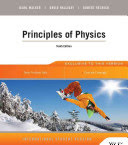### Physics 2

#### Learning Outcomes

1. Apply calculus techniques (derivative, integration) to analysis of physical problems.
2. Identify degrees of freedom of a rigid body.
3. Calculate moments of inertia for simple bodies.
4. Explain conditions for static equilibrium and the equation of motion for rotation of rigid body around a fixed axis.
5. Apply the equation of continuity and Bernoulli equation to simple problems in fluid mechanics.
6. Demonstrate the relationship between the microscopic and macroscopic variables in description of ideal gas.
7. Use the first law of thermodynamics in the analysis of thermodynamic processes.
8. Solve Schroedinger equation for 1D problems
9. Explain structure of semiconductors and the origin of energy bands
10. Explain electrical and magnetic properties of materials.

#### Forms of Teaching

Lectures

Lectures are delivered to student groups using electronic presentations, detailed derivations on the blackboard and demonstration experiments.

Partial e-learning

Assignments through e-learning platform

Laboratory

Student performed laboratory experiments

#### Grading Method

Continuous Assessment Exam
Type Threshold Percent of Grade Threshold Percent of Grade
Laboratory Exercises 5 % 10 % 5 % 10 %
Homeworks 0 % 10 % 0 % 10 %
Mid Term Exam: Written 0 % 40 % 0 %
Final Exam: Written 0 % 40 %
Exam: Written 0 % 80 %
##### Comment:

In the mid-term exam and in the final exam one (of four) problem must be correctly solved. In the written exam two (of six) problems must be correctly completed.

#### Week by Week Schedule

1. Solid body as the system of particles; Statics of the solid body; Moment of inertia of the solid body.
2. Rotation around the fixed axis (kinetic energy and angular momentum); Equation of motion for the rotation around fixed axis; General motion of the solid body (motion of CM with rotation around an axis).
3. Fluid statics; Dynamics of the perfect fluid; Viscosity in fluids.
4. Pressure and the mean kinetic energy of a gas particle; Equation of state; Degrees of freedom of the molecule; Thermal capacities of gases; Maxwell distribution of particle velocities; Equipartition of energy and Maxwell-Boltzmann distribution.
5. Heat; Temperature; Thermal equilibrium; Thermal expansion of solids and fluids; Equation of state of the ideal gas; Calorimetry; Thermal capacities; Phase transitions; Latent heats; Heat transport by convection; Heat transport by conduction; Fourier law; Heat flow in cylindrical and spherical symmetry; Heat transport by radiation; Black body; Stefan-Boltzmann law.
6. Work, heat and internal energy; First law of thermodynamics; Functions of state and of process; Isothermal, isobaric, isochoric and adiabatic processes; PV diagrams; Thermal capacities at constant volume and at constant pressure; Mayer's relation.
7. Carnot process; Efficiency of the heat engine; Second law of thermodynamics; Inverse Carnot process; Efficiency of the heat pump and the refrigerator; Entropy; Carnot process in the TS diagram.
8. Midterm exam.
9. Wave-particle duality; Quantum mechanics: postulates, epistemology, derivation, and solutions of the Schrödinger equation.
10. Properties and interpretation of a wave function; Bound states and electron orbitals.
11. Kronig–Penney model; Bloch theorem.
12. Fermi–Dirac distribution and its applications; Free electron model; Fermi energy; Electron state density function; Brillouin zones; Fermi energy; Fermi temperature; Fermi surface; Metal conductivity.
13. Quantum theory of semiconductors; Intrinsic semiconductors and heterogeneous structures; Effective mass of electrons and holes; Experimental measurements of the effective mass.
14. Classical and quantum theory of polarization; Diamagnetism, paramagnetism and ferromagnetism.
15. Final exam.

#### Study Programmes

##### University undergraduate
Electrical Engineering and Information Technology (study)
(3. semester)

#### Literature

Dubravko Horvat (2005.), Fizika 1: Mehanika i toplina, Hinus
Dubravko Horvat (2011.), Fizika 2: titranje, valovi, elektromagnetizam, optika i uvod u modernu fiziku, Neodidacta, Zagreb
Tomislav Petković (2016.), Uvod u znanost o toplini i termodinamici, Element
Petar Kulišić (2005.), Mehanika i toplina, Školska knjiga, Zagreb
Višnja Henč-Bartolić, Petar Kulišić (1991.), Valovi i optika, Školska knjiga, ZagrebDavid Halliday, Robert Resnick, Jearl Walker (2014.), Principles of Physics, J. Wiley
V. Henč-Bartolić, M. Baće. P. Kulišić, L. Bistričić, D. Horvat, Z. Narančić, T. Petković, D. Pevec (2002.), Riješeni zadaci iz valova i optike, Školska knjiga, Zagreb
V. Henč-Bartolić, M. Baće, L. Bistričić, D. Horvat, P. Kulišić, Z. Narančić, T. Petković, D. Pevec (1996.), Riješeni zadaci iz mehanike i topline, Školska knjiga, zagreb

#### General

ID 183395
Winter semester
6 ECTS
L3 English Level
L1 e-Learning
75 Lectures
0 Exercises
6 Laboratory exercises
0 Project laboratory

85 Excellent
70 Very Good
60 Good
50 Acceptable